Electron. J. Diff. Eqns., Vol. 2003(2003), No. 77, pp. 1-14.

### The Kolmogorov equation with time-measurable coefficients Jay Kovats

Abstract:
Using both probabilistic and classical analytic techniques, we investigate the parabolic Kolmogorov equation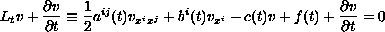in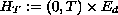and its solutions when the coefficients are bounded Borel measurable functions of. We show that the probabilistic solution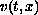defined in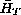, is twice differentiable with respect to, continuously in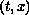, once differentiable with respect to, a.e.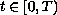and satisfies the Kolmogorov equation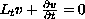a.e. in. Our main tool will be the Aleksandrov-Busemann-Feller Theorem. We also examine the probabilistic solution to the fully nonlinear Bellman equation with time-measurable coefficients in the simple case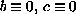. We show that when the terminal data function is a paraboloid, the payoff function has a particularly simple form.

Submitted March 11, 2003. Published July 13, 2003.
Math Subject Classifications: 35K15, 35B65, 35K15, 60J60.
Key Words: Diffusion processes, Kolmogorov equation, Bellman equation.

Show me the PDF file (245K), TEX file, and other files for this article.

Jay Kovats
Department of Mathematical Sciences
Florida Institute of Technology
Melbourne, FL 32901, USA
email: jkovats@zach.fit.edu